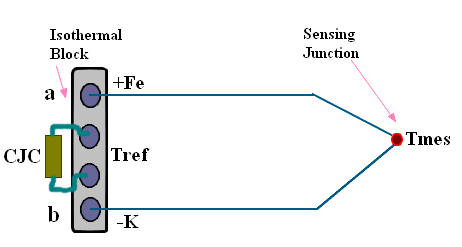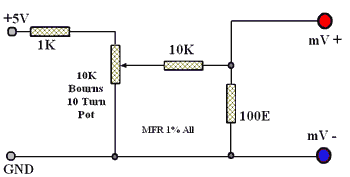## Solderman Basic Electronics

Basic Electronics, Practical Electronic Projects, Learning Circuit design, Electrical Fundamentals. Edutainment and Interactive Examples.

## Tuesday, January 06, 2009

### Thermocouple Temperature using DPM or DMM

#### Description -

If wires of two dissimilar metals are joined at both the ends and the junction formed at one of the ends, is heated more than the other junction, a current flows in the circuit due to Seebeck thermal emf. This effect is used in thermocouple temperature sensors.

The Peltier effect is the converse of above Seebeck effect, which means that if a current is forced through junctions of dissimilar metals, the junction will generate heat or absorb heat (cooling) depending on direction of the applied emf. This effect is used to make portable and small refrigerators.

Going to practical temperature measurement, we know that one of the junctions is the sensing or hot junction (Tmes) and the other junction is the terminating or cold junction (Tref), the voltage between terminals 'a' and 'b' is proportional to Tmes - Tref (and given in the Table 1) . The formula being Vab = alpha x (Tmes - Tref), where 'alpha' is the Seebeck coefficient of the thermocouple.#### Table 1

 MV Thermocouple Temperature in Deg C As cold junction is not zero but is at room temperature (RT) add RT to temperature. 0 0 2.585 50 5.268 100 10.777 200 Example - Feed 10.777 mV to the TC+ and TC- terminal if RT then is 30 Deg C reading on 2V DPM Will be 230 counts - 230mV. 16.325 300 21.846 400 27.338 500 33.096 600 Reference junction or cold junction at 0 deg C.

In the circuit, use only metal film resistors (MFR) of 1 per cent tolerance, as this is an instrumentation application. Power supply should be a stable +5V, -5V supply, for which one can use 7805 and 7905 regulators.

The inputs TC+ and TC- terminals should go to a 4-way barrier terminal block, the 2 extra terminals are used to mount TH1 Cu thermistor. This forms an isothermal block, which is good enough.

A simple way to make a TH1 Cu thermistor, is to take a 1 Meg-ohm 2W resistor as a former and wind 2 meters of 46 SWG enameled copper (Cu) wire (5.91 ohm/meter) over it. This gives a 12-ohm value. Terminate wire ends on resistor leads.

#### Circuit Diagram -

Thermocouple Amplifier Circuit - PDF version of above, more details and easily printable.

#### Test and Calibration -

For calibration, you will need a DMM-DPM and a milli-volt source (as shown in the Fig.). First connect source to terminals TC+ and TC-, then set source to 0.00 mV (verify with DMM for zero). The output across +out and -out (use DMM) terminals must be mV representing the room temperature (RT). For example, if RT is 30° C (use a glass thermometer) then +out should be 30mV at 0mV input. Adjust VR1 till 30mV is read at +out terminal. This is 'zero cal'.Now increase mV input to 21.85 (corresponding to 400° C). Now vary VR2 till +out terminal is at 430mV (temp. +RT). This is 'gain cal'. Now as VR1 and VR2 are interdependent you may have to repeat 'zero cal' and 'gain cal' a few times till you get the above values.

Properties of J thermocouple and design aspects of gain block used in the temperature measurement instrument are summarized below:

#### J Thermocouple Ansi Symbol 'J' -

1. J is a thermocouple made of iron + VE and constantan -VE.
2. Constantan is an alloy of copper and nickel.
3. Full range of use is from -200° to +700°C
4. Practical to use only from 0°C to 400°C.
5. Useful in reducing and Alkaline atmosphere.
6. Corrodes-rusts in acidic and oxidizing atmosphere
7. Color code of wires negative-red and positive-white.
8. J type is popular because of Low price and high mV output.
9. J type TC used in rubber-plastic forming and general purpose use.

#### Design of Gain Block -

1. Minimum input from thermocouple is very low like 1-2 mV. Hence ultra low offset (100uV opamp is required - OP07 used).
2. Inputs may be subjected to wrong connections or high voltage. Use of R1 limits current and Zener ZD1 clamps voltage to safe level. (low leakage zener or use diode).
3. Gain required is 400mV - 21.8mV that is approx 18 at 400° C. Gain Av = ( Rf + Ri ) / Ri here Rf is R7 and Ri = R5 + R6 + VR2 (in circuit value).
Design of TH1 cold junction compensation copper thermistor -
J Type TC output changes by 0.052mV per deg C as per Table 1. Copper has a temperature coefficient of 0.0042 ohm per ohm/deg C. eg. for a copper wire of 12 ohms, it is 12 x 0.0042 = 0.05 ohm/deg C.

For R1 of 5K current Thru TH1 =5V / 5K = 1mA. Change of voltage across TH1 with temperature is
0.05 x 1mA = 0.05mV / deg. This rate is same as J type TC hence it simulates cold junction

delabs Technologies